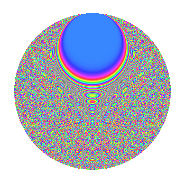# Properties

 Label 3150.2.cqLevel 3150 Weight 2 Character orbit cq Rep. character $$\chi_{3150}(331,\cdot)$$ Character field $$\Q(\zeta_{15})$$ Dimension 1920 Sturm bound 1440

# Learn more about

## Defining parameters

 Level: $$N$$ = $$3150 = 2 \cdot 3^{2} \cdot 5^{2} \cdot 7$$ Weight: $$k$$ = $$2$$ Character orbit: $$[\chi]$$ = 3150.cq (of order $$15$$ and degree $$8$$) Character conductor: $$\operatorname{cond}(\chi)$$ = $$1575$$ Character field: $$\Q(\zeta_{15})$$ Sturm bound: $$1440$$

## Dimensions

The following table gives the dimensions of various subspaces of $$M_{2}(3150, [\chi])$$.

Total New Old
Modular forms 5824 1920 3904
Cusp forms 5696 1920 3776
Eisenstein series 128 0 128

## Trace form

 $$1920q$$ $$\mathstrut +\mathstrut 240q^{4}$$ $$\mathstrut +\mathstrut O(q^{10})$$ $$1920q$$ $$\mathstrut +\mathstrut 240q^{4}$$ $$\mathstrut +\mathstrut 26q^{15}$$ $$\mathstrut +\mathstrut 240q^{16}$$ $$\mathstrut +\mathstrut 12q^{17}$$ $$\mathstrut -\mathstrut 16q^{18}$$ $$\mathstrut +\mathstrut 12q^{21}$$ $$\mathstrut +\mathstrut 16q^{23}$$ $$\mathstrut -\mathstrut 96q^{26}$$ $$\mathstrut -\mathstrut 48q^{27}$$ $$\mathstrut -\mathstrut 14q^{30}$$ $$\mathstrut +\mathstrut 30q^{33}$$ $$\mathstrut +\mathstrut 14q^{35}$$ $$\mathstrut -\mathstrut 32q^{39}$$ $$\mathstrut +\mathstrut 32q^{41}$$ $$\mathstrut +\mathstrut 28q^{42}$$ $$\mathstrut -\mathstrut 50q^{45}$$ $$\mathstrut +\mathstrut 4q^{50}$$ $$\mathstrut -\mathstrut 12q^{51}$$ $$\mathstrut -\mathstrut 40q^{53}$$ $$\mathstrut +\mathstrut 12q^{55}$$ $$\mathstrut +\mathstrut 96q^{57}$$ $$\mathstrut +\mathstrut 24q^{58}$$ $$\mathstrut +\mathstrut 24q^{59}$$ $$\mathstrut -\mathstrut 2q^{60}$$ $$\mathstrut +\mathstrut 36q^{62}$$ $$\mathstrut +\mathstrut 16q^{63}$$ $$\mathstrut -\mathstrut 480q^{64}$$ $$\mathstrut +\mathstrut 10q^{65}$$ $$\mathstrut -\mathstrut 16q^{66}$$ $$\mathstrut +\mathstrut 96q^{68}$$ $$\mathstrut +\mathstrut 42q^{69}$$ $$\mathstrut -\mathstrut 24q^{70}$$ $$\mathstrut +\mathstrut 36q^{71}$$ $$\mathstrut +\mathstrut 8q^{72}$$ $$\mathstrut -\mathstrut 226q^{75}$$ $$\mathstrut -\mathstrut 112q^{77}$$ $$\mathstrut -\mathstrut 16q^{78}$$ $$\mathstrut -\mathstrut 12q^{79}$$ $$\mathstrut +\mathstrut 52q^{81}$$ $$\mathstrut +\mathstrut 84q^{83}$$ $$\mathstrut +\mathstrut 36q^{84}$$ $$\mathstrut +\mathstrut 88q^{87}$$ $$\mathstrut +\mathstrut 18q^{89}$$ $$\mathstrut +\mathstrut 88q^{90}$$ $$\mathstrut +\mathstrut 12q^{92}$$ $$\mathstrut -\mathstrut 116q^{93}$$ $$\mathstrut -\mathstrut 34q^{95}$$ $$\mathstrut +\mathstrut 48q^{98}$$ $$\mathstrut +\mathstrut 32q^{99}$$ $$\mathstrut +\mathstrut O(q^{100})$$

## Decomposition of $$S_{2}^{\mathrm{new}}(3150, [\chi])$$ into irreducible Hecke orbits

The newforms in this space have not yet been added to the LMFDB.

## Decomposition of $$S_{2}^{\mathrm{old}}(3150, [\chi])$$ into lower level spaces

$$S_{2}^{\mathrm{old}}(3150, [\chi]) \cong$$ $$S_{2}^{\mathrm{new}}(1575, [\chi])$$$$^{\oplus 2}$$